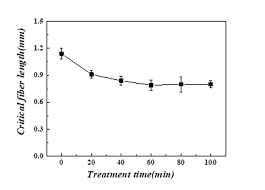## How to Calculate and Solve for Critical Fibre Length | Composites

The critical fibre length is illustrated by the image below.To compute for critical fibre length, three essential parameters are needed and these parameters are Critical Fibre Stress (σ*f), Fibre Diameter (d) and Shear Yield Strength (τc).

The formula for calculating critical fibre length:

lc = σ*fd/2τc

Where:

lc = Critical Fibre Length
σ*f = Critical Fibre Stress
d = Fibre Diameter
τc = Shear Yield Strength

Let’s solve an example;
Find the critical fibre length when the critical fibre stress is 15, the fibre diameter is 3 and the shear yield strength is 10.

This implies that;

σ*f = Critical Fibre Stress = 15
d = Fibre Diameter = 3
τc = Shear Yield Strength = 10

lc = σ*fd/2τc
lc = (15)(3)/2(10)
lc = (45)/(20)
lc = 2.25

Therefore, the critical fibre length is 2.25 cm.

Calculating the Critical Fibre Stress when the Critical Fibre Length, the Fibre Diameter and the Shear Yield Strength is Given.

σ*f = lc x 2τc / d

Where:

σ*f = Critical Fibre Stress
lc = Critical Fibre Length
d = Fibre Diameter
τc = Shear Yield Strength

Let’s solve an example;
Given the critical fibre length as 24, the fibre diameter as 12 and the shear yield strength as 4. Find the critical fibre stress?

This implies that;

lc = Critical Fibre Length = 24
d = Fibre Diameter = 12
τc = Shear Yield Strength = 4

σ*f = lc x 2τc / d
σ*f = 24 x 2(4) / 12
σ*f = 24 x 8 / 12
σ*f = 192 / 12
σ*f = 16

Therefore, the critical fibre stress is 16.